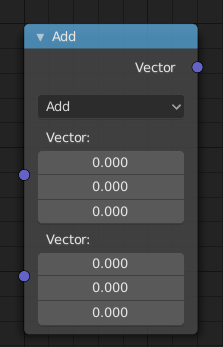# Vector Math Node¶Vector Math Node.

The Vector Math node performs the selected math operation on the input vectors.

## Inputs¶

The inputs of the node are dynamic. Some inputs are only available in certain operations. For instance, the Scale input is only available in the Scale operator.

Vector
Input vector A.
Vector
Input vector B.
Scale
Input Scale.

## Properties¶

Operation

The vector math operator to be applied on the input vectors.

The sum of A and B.
Subtract
The difference between A and B.
Multiply
The entrywise product of A and B. $$(A.x * B.x, A.y * B.y, A.z * B.z)$$
Divide
The entrywise division of A by B. Division by zero returns zero. $$(A.x / B.x, A.y / B.y, A.z / B.z)$$
Cross Product
The cross product of A and B.
Project
The projection of A onto B.
Reflect
The reflection of A around the normal B. B need not be normalized.
Dot Product
The dot product of A and B.
Distance
The distance between A and B.
Length
The length of A.
Scale
The result of multiplying A by the scalar input Scale.
Normalize
The result of normalizing A.
Snap
The result of rounding A to the largest integer multiple of B less than or equal A.
Floor
The entrywise floor of A.
Ceil
The entrywise ceiling of A.
Modulo
The entrywise modulo of A by B.
Fraction
The fractional part of A.
Absolute
The entrywise absolute value of A.
Minimum
The entrywise minimum from A and B.
Maximum
The entrywise maximum from A and B.

## Outputs¶

The output of the node is dynamic. It is either a vector or a scalar depending on the operator. For instance, the Length operator have a scalar output while the Add operator have a vector output.

Vector
Output vector.
Value
Output value.